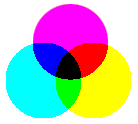Home : Lighting : Colour : Subtractive Primaries

# Subtractive PrimariesThe subtractive primary colours are cyan, yellow and magenta. These colours are used in print media for dye and pigments. They are distinct from the additive primaries used when considering light sources and RGB colour models.

In subtractive primary colours:

• Cyan + Magenta = Blue
• Magenta + Yellow = Red
• Yellow + Cyan = Green
• Cyan + Magenta + Yellow = Black

Subtractive primaries are so-called because they subtract (absorb) certain colours from the light falling upon them. This is how objects appear to be coloured — if you shine white light on them, they absorb certain frequencies of the light and the reflected frequencies create a colour.

• A red object subtracts green and blue (cyan) light
• A green object subtracts red and blue (magenta) light
• A blue object subtracts green and red (yellow) light
• A magenta object subtracts green light
• A cyan object subtracts red light
• A yellow object subtracts blue light